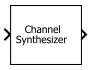# Channel Synthesizer

Polyphase FFT synthesis filter bank

• Library:
• DSP System Toolbox / Filtering / Multirate Filters

•## Description

The Channel Synthesizer block merges multiple narrowband signals into a broadband signal by using an FFT-based synthesis filter bank. The filter bank uses a prototype lowpass filter and is implemented using a polyphase structure. You can specify the filter coefficients directly or through design parameters. When you specify the design parameters, the filter is designed using the `designMultirateFIR` function.

This block also accepts variable-size inputs. That is, during the simulation, you can change the size of each input channel. The number of channels cannot change.

## Ports

### Input

expand all

Input narrowband signals, which the channel synthesizer merges to form the broadband signal. Each narrowband signal forms a column in the input signal. The number of columns in the input correspond to the number of frequency bands of the filter bank. If the input is three-dimensional, each matrix corresponds to a separate channel.

This port is unnamed until you set Polyphase filter specification to `Coefficients` and select the Specify coefficients from input port parameter.

Data Types: `single` | `double`
Complex Number Support: Yes

Coefficients of the prototype lowpass filter. There must be at least one coefficient per frequency band. If the length of the lowpass filter is less than the number of frequency bands, the block zero-pads the coefficients.

If you specify complex coefficients, the block designs a prototype filter that is centered at a nonzero frequency, also known as a bandpass filter. The modulated versions of the prototype bandpass filter appear with respect to the prototype filter and are wrapped around the frequency range [−Fs Fs].

#### Dependencies

This port appears when you set Polyphase filter specification to `Coefficients` and select the Specify coefficients from input port parameter.

Data Types: `single` | `double`
Complex Number Support: Yes

### Output

expand all

Broadband signal the channel synthesizer forms from the multiple input narrow subbands.

If the input is one of the following:

• L-by-M matrix — The output is a L×M-by-1 vector. M is the number of frequency bands.

• L-by-M-by-N matrix — The output is a L×M-by-N matrix.

Data Types: `single` | `double`
Complex Number Support: Yes

## Parameters

expand all

If a parameter is listed as tunable, then you can change its value during simulation.

• ```Number of taps per band and stopband attenuation``` — Specify the filter design parameters through the Number of filter taps per frequency band and Stopband attenuation (dB) parameters. When you specify the design parameters, the filter is designed using the `designMultirateFIR` function.

• `Coefficients` — Specify the filter coefficients directly using the Prototype lowpass filter coefficients parameter or input them through the coeffs port.

Number of filter coefficients that each polyphase branch uses. The number of polyphase branches matches the number of frequency bands. The total number of filter coefficients for the prototype lowpass filter is given by the product of the number of frequency bands and the number of filter taps per frequency band. The number of frequency bands equals the number of columns in the input. For a given stopband attenuation, increasing the number of taps per band narrows the transition width of the filter. As a result, there is more usable bandwidth for each frequency band, at the expense of increased computation.

#### Dependencies

To enable this parameter, set Polyphase filter specification to ```Number of taps per band and stopband attenuation```.

Stopband attenuation of the lowpass filter, in dB. This value controls the maximum amount of aliasing from one frequency band to the next. As the stopband attenuation increases, the passband ripple decreases.

#### Dependencies

To enable this parameter, set Polyphase filter specification to ```Number of taps per band and stopband attenuation```.

When you select this check box, the lowpass filter coefficients are input through the coeffs port. When you clear this check box, the coefficients are specified on the block dialog through the Prototype lowpass filter coefficients parameter.

#### Dependencies

To enable this parameter, set Polyspace filter specification to `Coefficients`.

This parameter specifies the coefficients of the prototype lowpass filter. The default value is the coefficients vector that `rcosdesign(0.25,6,8,'sqrt')` returns. There must be at least one coefficient per frequency band. If the length of the lowpass filter is less than the number of frequency bands, the block zero-pads the coefficients.

If you specify complex coefficients, the block designs a prototype filter that is centered at a nonzero frequency, also known as a bandpass filter. The modulated versions of the prototype bandpass filter appear with respect to the prototype filter and are wrapped around the frequency range [−Fs Fs].

Tunable: Yes

#### Dependencies

To enable this parameter, set Polyphase filter specification to `Coefficients` and clear the Specify coefficients from input port parameter.

Complex Number Support: Yes

• `Interpreted execution`

Simulate model using the MATLAB® interpreter. This option shortens startup time and has faster simulation speed compared to ```Code generation```.

• `Code generation`

Simulate model using generated C code. The first time you run a simulation, Simulink® generates C code for the block. The C code is reused for subsequent simulations, as long as the model does not change. This option requires additional startup time but provides faster subsequent simulations.

## Block Characteristics

 Data Types `double` | `single` Multidimensional Signals `No` Variable-Size Signals `Yes`

expand all

## Algorithms

expand all

 Harris, Fredric J, Multirate Signal Processing for Communication Systems, Prentice Hall PTR, 2004.

 Harris, F.J., Chris Dick, and Michael Rice. "Digital Receivers and Transmitters Using Polyphase Filter Banks for Wireless Communications." IEEE® Transactions on Microwave Theory and Techniques. Vol. 51, Number 4, April 2003.

## SupportGet trial now